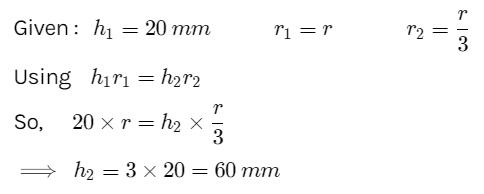Courses

# Test: Surface Tension & Capillarity

## 10 Questions MCQ Test Physics For JEE | Test: Surface Tension & Capillarity

Description
This mock test of Test: Surface Tension & Capillarity for JEE helps you for every JEE entrance exam. This contains 10 Multiple Choice Questions for JEE Test: Surface Tension & Capillarity (mcq) to study with solutions a complete question bank. The solved questions answers in this Test: Surface Tension & Capillarity quiz give you a good mix of easy questions and tough questions. JEE students definitely take this Test: Surface Tension & Capillarity exercise for a better result in the exam. You can find other Test: Surface Tension & Capillarity extra questions, long questions & short questions for JEE on EduRev as well by searching above.
QUESTION: 1

### The angle of contact for liquid on a solid surface is the angle between:

Solution:

The angle of contact for liquid on a solid surface is the angle between the tangent to the liquid surface at the point of contact and the solid surface. This is the definition of angle of contact.

QUESTION: 2

### When impurity is added to a liquid, its surface tension

Solution:

When impurities are mixed in liquid, the surface tension of liquid decreases. The soluble substances when dissolved in water, decreases the surface tension of water.

QUESTION: 3

### If drops and bubbles do not collapse under the effect of gravity, it indicates that

Solution:

If drops and bubbles do not collapse under the effect of gravity, it indicates that the pressure inside the drop is greater than the pressure outside. The greater inner pressure prevents the drop from collapsing.

QUESTION: 4

By which phenomenon does the water rise from roots to leaves of plants?

Solution:

Plants use capillary action to bring water from the soil up through capillaries, small tubes in the plants, to the rest of the plant. Due to gravity, capillary action is not strong enough for the water to travel to the top of the plant. Another process, transpiration pull does the rest of the work.

QUESTION: 5

When a capillary tube of radius r is dipped in a liquid of density ρ and surface tension S, the liquid rises or falls through a distance

Solution:
QUESTION: 6

The excess pressure inside an air bubble is

Solution:
QUESTION: 7

SI unit of surface tension is

Solution:

Surface tension is defined as the ratio of surface force applied on a liquid to the length along which the force acts. so it is newton/meter

QUESTION: 8

When an air bubble of radius R lies at a depth h below the free surface of a liquid of density ρ and surface tension Sla, then the excess pressure inside the bubble will be

Solution:

Excess pressure inside a cavity or air bubble in liquid formula
P[excess] = P[inside] - P [outside]
P [outside]  =P[atm]
P[inside]= P[atm] +hpg [here p represents density] +2T/R
P[excess] = P[atm] +hpg [here p represents density] +2T/R-P[atm]
P[excess] = hpg+2T/R
Now substitute value for T
P[excess] = hpg+2S/R
Hence C is correct.

QUESTION: 9

Water rises to a height of 20 mm in a capillary. If the radius of the capillary is made 1/3 rd of its previous value, to what height will the water now rise in the tube?

Solution:QUESTION: 10

The excess pressure inside a soap bubble is (Here, Sla is the surface tension between the liquid-air interface).

Solution:

Excess pressure in water bubble = 2T/r (due to 1 surface only)
Excess pressure in a soap bubble = 4T/r (due to 2 surfaces)